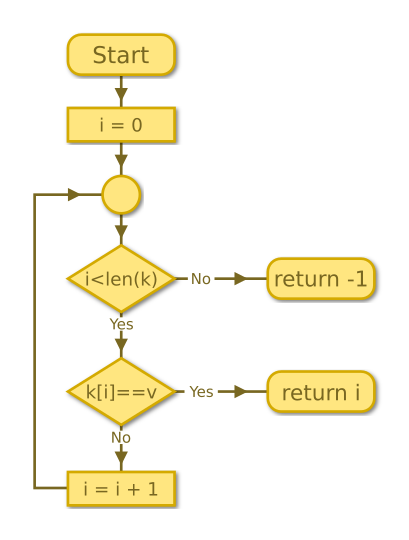# Linear search

Martin McBride, 2016-12-14
Tags linear search
Categories algorithms search

Suppose you have a set of cards, face down on the table. Each card has a number on it. The cards have been shuffled, so they are in a random order. You must find the card with a number 3 printed on it.## A simple linear search

How would you go about it? The best way is simply to start at the first card and work through them in order. We call this process a linear search.

Turn over the first card:That card isn't a 3, so try the next card:That isn't a 3 either, so try the next card:Finally we have found it, the card we were looking for is at position 2 (remember, programmers count positions from zero). Another example is shown in the video below:

## Defining the computer algorithm

This description of the algorithm is fine for a human reader, but it is not quite detailed enough for a computer to use. We need to define what inputs the algorithm has, and what the output will be in every possible case. Then we can create our pseudo code or flow chart to fully define the algorithm.

## Inputs

In the practical example above, we searched a set of cards for a particular value (which happened to be 3 in the example).

To represent the set of cards, we will use a list (or array) of integer values, which we will call k. For example:

k = [5, 8, 3, 2, 1, 4, 9]


The second input, v, is the value we are searching for.

## Outputs

When we search for value the v in the list k, we should return the position of the value in the list. The position is the zero based index, so for example:

v = 1
k = [5, 8, 3, 2, 1, 4, 9]


will result in a return value of 4 (because the 5th element in k has value 1, and the 5th element has index 4 when we count from zero).

There are two possibilities we haven't covered:

• The value v appears more than once in k - in that case, we will return the first position where value v occurs.
• The value v doesn't appear in k at all - in that case, we can return -1. This makes it easy for the code which uses the algorithm to detect the special case, and handle it properly.

## Pseudo code

Here is the algorithm in pseudo code:

INPUTS k, v
SET i = 0
WHILE i is less than the length of k
IF k[i] equals v
RETURN i
SET i = i + 1

RETURN -1

We use the variable i to point to the current value. i starts at 0 and counts up to one less than the length of the list. So if the list has 5 elements, i will count 0, 1, 2, 3, 4 (which are the indices of the 5 list elements).

If one of the elements matches v, the algorithm returns the current index and stops.

If a matching element is not found, the loop ends and the final line of pseudo code returns -1.

## FlowchartCopyright (c) Axlesoft Ltd 2021